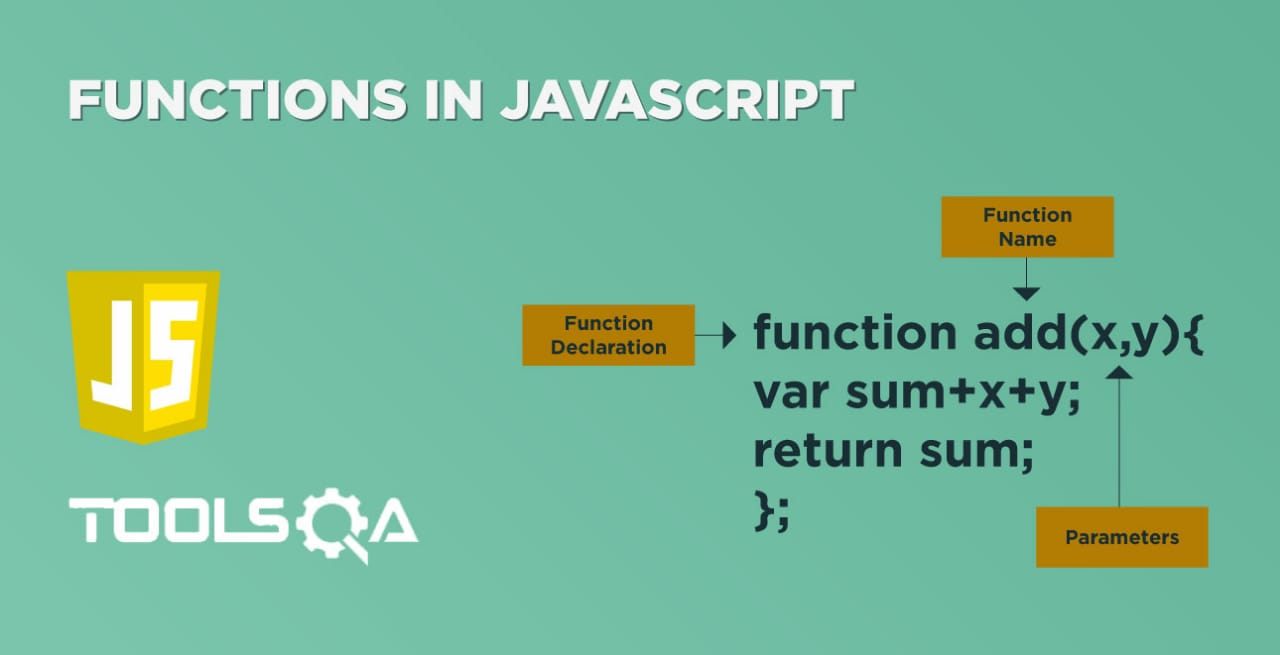Operators? Does it ring a bell? Yes, during our school/college days, we must come across the term "Operators". In mathematics, an operator refers to any symbol that indicates an operation that has to perform. In the same vein, it is no different when it comes to Javascript. We are going to talk in detail about Javascript operators in this article. Therefore, let's get started.

## What are JavaScript Operators?

Operators are the symbols or keywords which signifies the operation that needs to carry out on the operands. In other words, an operator is capable of either manipulating a particular value or producing a result by comparing or operating on the given values. Like other programming languages, JavaScript also supports multiple types of operators. We will be covering the following in this article:

• Arithmetic Operators
• Assignment Operators
• Comparison Operators
• Logical Operators
• Bitwise Operators
• Miscellaneous Operators

### Arithmetic Operators:

Arithmetic Operators are symbols that represent mathematical operations. Additionally, the arithmetic operator takes two operands and performs a calculation on them. JavaScript supports the following arithmetic operators:

Name Operator Syntax Example
Addition + Output = var1+var2; Output = 5+3;
Subtraction - Output = var1-var2; Output = 5-3;
Multiplication * Output = var1*var2; Output = 5*3;
Division / Output = var1/var2; Output = 5/3;
Modulus % Output = var1%var2; Output = 5%3;
Pre Increment ++ ++output var output = 5;++output;
Post Increment ++ output++ var output = 5;output++;
Pre Decrement - - --output var output = 5;- -output;
Post Decrement - - output-- var output = 5;output- -;

Note: As we can see from the "syntax" and "Example" column above, we use the Simple assignment operator (=) to assign the calculated value of the arithmetic operators to the operand. Moreover, this operator is used to assign the right-side value to the left-side variable. Additionally, we will cover the details of this operator in the next section of "Assignment Operators".

Now let's understand the Arithmetic as mentioned above operators in detail:

• Addition (+):  This operator provides the sum of two operands.
``````var a = 7;
var b = 5;
var result = a+b;//this will provide output as 12
``````
• Subtraction (-): This operator provides the difference of two operands.
``````var a = 7;
var b = 5;
var result = a-b;//this will provide output as 2
``````
• Multiplication ( * ): This operator provides a multiplied value of two operands.
``````var a = 7;
var b = 5;
var result = a*b;//this will provide output as 35

``````
• Division (/): This operator provides a divided value of two operands.
``````var a = 7;
var b = 5;
var result = a/b;//this will provide output as 1
``````
• Modulus (%): This operator provides a remainder value of two operands.
``````var a = 7;
var b = 5;
var result = a%b;//this will provide output as 2
``````
• Pre-Increment (++variable): This operator increments the integer value by one before its usage or assignment.
``````var a = 7;
var result = ++a;//in this step value of result=8 and value of a=8
``````
• Post-Increment (variable++): This operator will increment the integer value by one after its usage or assignment.
``````var a = 7;
var result = a++;//in this step value of result=7 and value of a=8
``````
• Pre-Decrement (--variable): This operator will decrement the integer value by one before its usage or assignment.
``````var a = 7;
var result = --a;//in this step value of result=6 and value of a=6

``````
• Post-Decrement (variable--): This operator will decrement the integer value by one after its usage or assignment.
``````var a = 7;
var result = a--;//in this step value of result=7 and value of a=6

``````

Let’s create a simple example using all the arithmetic as mentioned above operators:

``````<html>

<body>

Set the variables to different values and different arithmetic operators and then try...

<script type = "text/javascript">

var a = 7;

var b = 5;

var result;

var linebreak = "<br />";

document.write(linebreak);

document.write(`value of a = \${a} and value of b = \${b}`);

document.write(linebreak);

document.write(`(a = \${a})`);

document.write(linebreak);

document.write('(a + b) => ');

result = a+b;

document.write(result);

document.write(linebreak);

document.write('(b - a) => ');

result = a-b;

document.write(result);

document.write(linebreak);

document.write('(a * b) => ');

result = a*b;

document.write(result);

document.write(linebreak);

document.write('(a / b) => ');

result = a/b;

document.write(result);

document.write(linebreak);

document.write('(a % b) => ');

result = a%b;

document.write(a);

document.write(linebreak);

result = ++a;

document.write(`result = ++a => \${result} (result value) and \${a} (a value)`);

document.write(linebreak);

var a = 7;

result = a++;

document.write(`result = a++ => \${result} (result value) and \${a} (a value)`);

document.write(linebreak);

var a = 7;

result = --a;

document.write(`result = --a => \${result} (result value) and \${a} (a value)`);

document.write(linebreak);

var a = 7;

result = a--;

document.write(`result = a-- => \${result} (result value) and \${a} (a value)`);

document.write(linebreak);

</script>

</body>

</html>

``````

Open any text editors like notepad++. After that, type the above code. Save the file with name arithmeticOperators.html. Thereafter, open it in any browser (Chrome, Firefox or IE). It should show the output as: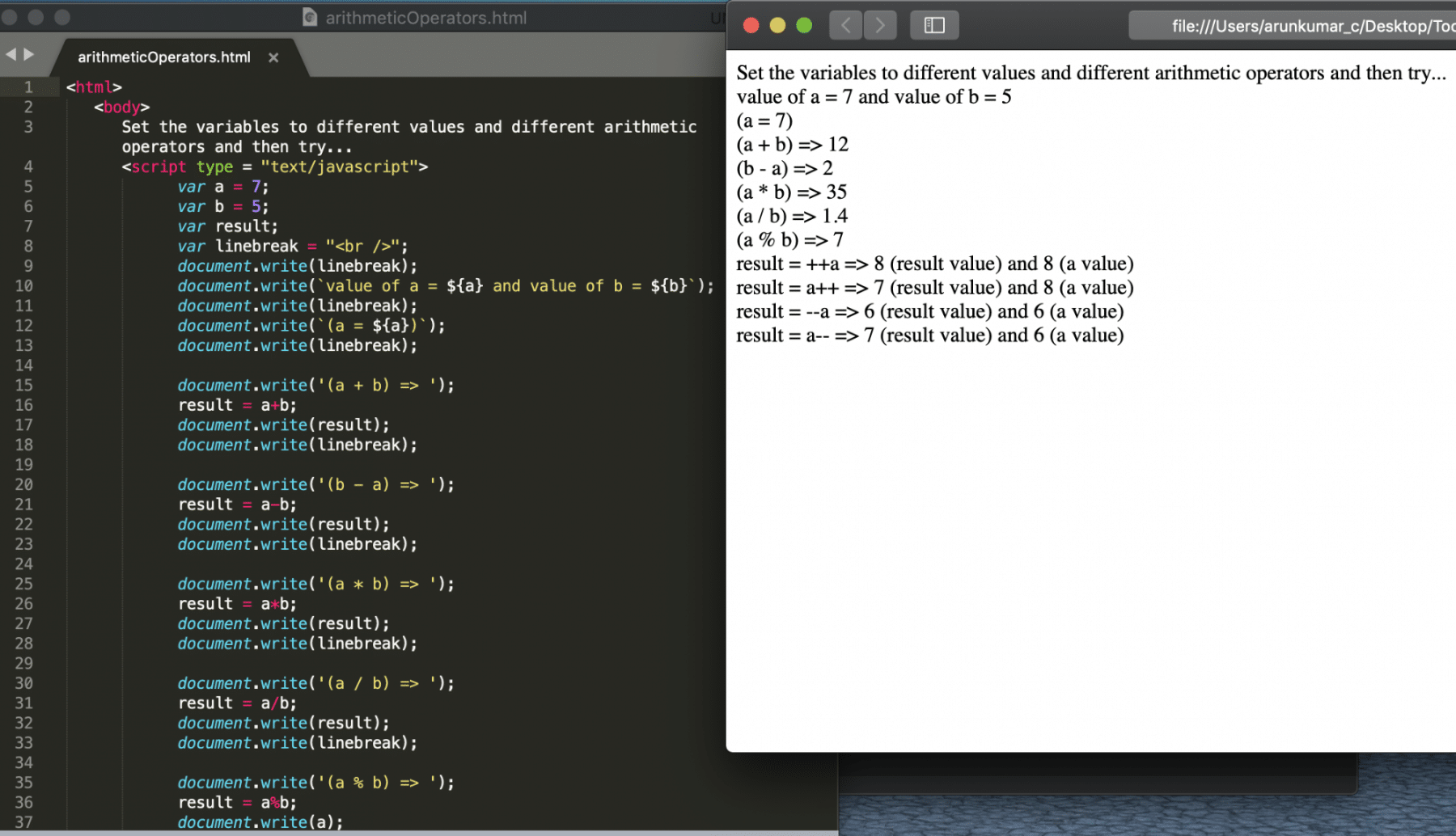### Assignment Operators:

Assignment operators are used to assigning values to a variable. Additionally, the left-hand side of the operator is a variable. And the right-hand side of the operator is the value that is going to be assigned to a variable. JavaScript supports the following assignment operators:

Name Operator Syntax Example
Simple Assignment = Output = var1; myVal = 3; output : 3
Addition and Assign += Output += var1; var myVal = 5; myVal += 5; output: 10
Subtraction and Assign -= Output -= var1; var myVal = 10; myVal -= 5; output: 5
Multipliy and Assign *= Output *= var1; var myVal = 5; myVal *= 5; output: 25
Divide and Assign /= Output /= var1; var myVal = 25; myVal /= 5; Output: 5
Modulus and Assign %= Output %= var1; var myVal= 54; myVal %= 5; output: 4

Now lets understand the above mentioned Assignment operators in detail.

• Simple Assignment ( = ): This operator assigns the right-side value to left-side variable.

Example:

``````var variableName = ‘Tools QA’;
``````
• Addition and Assign ( += ): This operator adds the value of the left-side variable with right-side value. Finally, it assigns the total to the left-side variable.

Example:

``````var sum = 5;
sum += 5; //The value of "sum" will be 10
``````
• Subtraction and Assign ( -= ): This operator subtracts the value of the right-side variable from left-side value. Finally, it assigns the subtracted value to the left-side variable.
``````var sub = 10;
sub -= 5;  // which is equivalent to sub = sub - 5; The value of "sub" will be 5 after this step
``````
• *Multiply and Assign ( = ): This operator multiplies the value of the left-side variable with right-side value. Consequently, it assigns the multiplied value to the left-side variable.
``````var mul = 5;
mul *=5; // which is equivalent to mul = mul * 5; The value of "mul" will be 25 after this step.
``````
• Divide and Assign ( /= ): This operator divides the value of the left-side variable with the right-side value. Finally, it assigns the divided value to the left-side variable.
``````var div = 25;
div /= 5; // which is equivalent to div = div / 5; The value of "div" will be 5 after this step.
``````
• Modulus and Assign ( %= ): This operator finds the modulus of the value of the left-side variable with right-side value. Thereafter, it assigns the remainder value to the left-side variable.
``````var div = 27;
div %= 5; // which is equivalent to div = div % 5; The value of "fiv" would be 2 after this step.
``````

Let’s create a simple example using above all the assignment operators.

``````<html>

<body>

Set the variables to different values and different assignment operators and then try...

<script type = "text/javascript">

var a = 5;

var b = 10;

var linebreak = "<br />";

document.write(linebreak); // This will just print a line break between the statements.

document.write(`value of a = \${a} and value of b = \${b}`);

document.write(linebreak);

document.write(`(a = \${a})`);

document.write(linebreak);

document.write('(a += b) => ');

a += b; //equivalent to a =a+b;

document.write(a);

document.write(linebreak);

a = 5;

b = 10;

document.write('(b -= a) => ');

b -= a; //equivalent to b = b-a;

document.write(b);

document.write(linebreak);

a = 5;

b = 10;

document.write('(a *= b) => ');

a *= b; //equivalent to a = a*b

document.write(a);

document.write(linebreak);

a = 5;

b = 10;

document.write('(b /= a) => ');

b /= a;//equivalent to b = b/a

document.write(b);

document.write(linebreak);

a = 5;

b = 10;

document.write('(a %= b) => ');

a %= b;

document.write(a);

document.write(linebreak);

</script>

</body>

</html>

``````

Save the file with name assignmentOperators.html. After that, open it in a browser.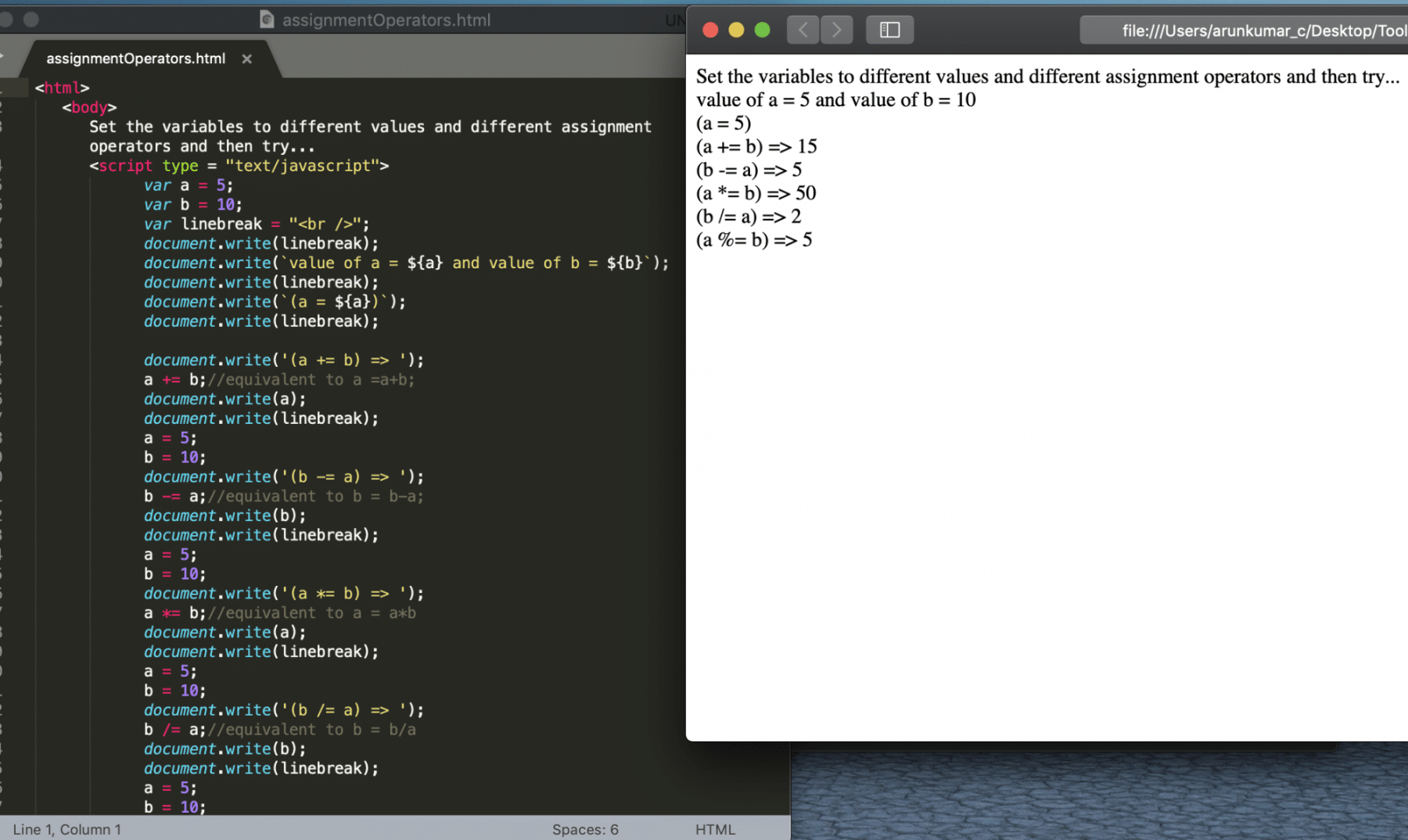### Comparison Operators:

Comparison operators are used to comparing the values of two operands. For Example: Validating if operand1 value is equal to operand2, or if operand1 value is greater than operand2, etc. In any case, the result of a comparison operator will always be a boolean value. In other words, either true or false.  JavaScript supports the following comparison operators:

Name Operator Syntax Example
Equals to == output = var1==var2; Output = 3==3;
Not Equals To != Output = var1!=var2; Output = 5!=4;
Greater Than > Output = var1>var2; Output = 5>4;
Greater Than or Equal To >= Output = var1>=var2; Output = 5>=5;
Lesser Than < Output = var1<var2; Output = 4<6;
Lesser Than or Equal To <= Output = var1<=var2; Output = 4<=4;

Now let's understand the Comparison as mentioned above operators in detail.

• Equals to ( == ): This operator compares whether operand1 value is equal to operand2. If given condition satisfies, then the result will be true, otherwise false. E.g., Consider below code-snippet:
``````var A = 5;
var B = 5;
var C = 7;
var out1 = (A == B); //Since A's value (5) is equal to B's value (5), out1 will be true
var out2 = (A == C); //Since A's value (5) is not equal C's value (7), out2 will be false
``````
• Not Equals To (!=):  This operator compares whether operand1 value is not equal to operand2. If given condition satisfies, then the result will be true, otherwise false. E.g., Consider below code-snippet:
``````var A = 5;
var B = 5;
var C = 7;
var out1 = (A != B); //Since A's value (5) is equal B's value (5), out1 will be false
var out2 = (A != C); //Since A's value (5) is not equal C's value (7), out2 will be true
``````
• Greater Than(>):  This operator compares whether the operand1 value is greater than operand2. If given condition satisfies then, the result will be true; otherwise, false. E.g., Consider below code-snippet:
``````var A = 6;
var B = 5;
var C = 7;
var out1 = (A > B); //Since A's value (6) is greater thanl B's value (5), out1 will be true
var out2 = (A > C); //Since A's value (6) is not greater than C's value (7), out2 will be false
``````
• Greater Than or Equal To (>=):  This operator compares whether operand1 value is greater than or equal to operand2. If given condition satisfies, then the result will be true, otherwise false. E.g., Consider below code-snippet:
``````var A = 6;
var B = 5;
var C = 6;
var D = 7;
var out1 = (A >= B); //Since A value (6) is greater thanl B value (5), out1 will be true
var out2 = (A >= C); //Since A value (6) is equal to C value (6), out2 will be true
var out3 = (A >= D); //Since A value (6) is not greater than or equal D value (7), out3 will be false
``````
• Lesser Than(<):  *This operator compares whether operand1 value is lesser than operand2. If given condition satisfies, then the result will be true, otherwise false. E.g., Consider below code-snippet:
``````var A = 4;
var B = 5;
var C = 3;
var out1 = (A < B); //Since A value (4) is lesser than B value (5), out1 will be true
var out2 = (A < C); //Since A value (4) is not lesser than C value (3), out2 will be false
``````
• Lesser Than or Equal To (<=):  This operator compares whether operand1 value is lesser than or equal to operand2. If given condition satisfies, then the result will be true, otherwise false. E.g., Consider below code-snippet:
``````var A = 6;
var B = 7;
var C = 6;
var D = 5;
var out1 = (A <= B); //Since A value (6) is lesser than B value (7), out1 will be true
var out2 = (A <= C); //Since A value (6) is equal to C value (6), out2 will be true
var out3 = (A <= D); //Since A value (6) is not lesser than or equal D value (5), out3 will be false
``````

For instance, let’s create a simple example using above all the comparison operators.

``````<html>

<body>

Set the variables to different values and different comparsions operators and then try...

<script type = "text/javascript">

var a = 7;

var b = 4;

var c = 7;

var d = 3;

var linebreak = "<br />";

document.write(linebreak);

document.write(`(\${a} == \${c}) => `);

result = (a == c);

document.write(result);

document.write(linebreak);

document.write(`(\${d} < \${b}) => `);

result = (d < b);

document.write(result);

document.write(linebreak);

document.write(`(\${a} > \${b}) => `);

result = (a > b);

document.write(result);

document.write(linebreak);

document.write(`(\${a} != \${b}) => `);

result = (a != b);

document.write(result);

document.write(linebreak);

document.write(`(\${a} >= \${c}) => `);

result = (a >= c);

document.write(result);

document.write(linebreak);

document.write(`(\${a} <= \${c}) => `);

result = (a <= b);

document.write(result);

document.write(linebreak);

</script>

</body>

</html>

``````

Save the file with name comparisonOperators.html. After that, open it in any browser.### Logical Operators:

Logical operators are primarily used to control program flow. Usually, you will find them as part of an if, while, or some other conditional statement. Additionally, explanation and more details of the conditional statement will come in later tutorials. The concept of logical operators is simple. They enable a program to make a decision based on multiple conditions. In other words, each operand is considered a condition that can evaluate to a true or false value.

JavaScript supports the following logical operators:

Name Operator Syntax Example
AND Operator && output = var1&&var2; Output = true&&true;
OR Operator || Output = var1||var2; Output = false||true;
NOT Operator ! Output = !var1; Output = !false

Now let's understand the above mentioned Logical operators in detail:

• AND Operator (&&): If both operands opr1 and opr2 are true, only then the condition will become true else it will be false. Additionally, it follows the simple Truth table, as shown below:
Operand1 Operand2 Operand1 AND Operand2
TRUE TRUE TRUE
TRUE FALSE FALSE
FALSE TRUE FALSE
FALSE FALSE FALSE

The logic can be illustrated and validated with the help of the following code snippet:

``````var a = true;
var b = false;
var c = true;
var out1 = a && c; // in this case value of out1 will be true since a and c values are true
var out2 = a && b; // in this case, the value of out2 will be false since any one of the values of a and be is false i.e, the value of b is false

``````
• OR Operator (||): If either of operands opr1 or opr2 is true, only then the condition will become true else it will be false. In addition to this, it follows the simple Truth table, as shown below:
Operand1 Operand2 Operand1 OR Operand2
TRUE TRUE TRUE
TRUE FALSE TRUE
FALSE TRUE TRUE
FALSE FALSE FALSE

The logic can be illustrated and validated with the help of the following code snippet:

``````var a = false;
var b = true;
var c = false;
var out1 = a || b; // in this case value of out1 will be true, since either one of the values of a and b is true
var out2 = a || c; // in this case value of out2 will be false, since both operands are false
``````
• NOT Operator ( ! ): Invert the boolean value of the operand. In other words, if the operand value is true, then the inverted value will be false and vice versa. Additionally, it is a unary operator, which means that it works on a single operand. It follows the simple Truth table, as shown below
Operand NOT Operand
TRUE FALSE
FALSE TRUE

The logic can be illustrated and validated with the help of the following code snippet:

``````var a = true;
var b = false;
var out1 = !a; //value of out1 will be false
var out2 = !b; //value of out2 will be true
``````

Let’s create a simple example using all the above logical operators:

``````<html>

<body>

Set the variables to different values and different logical operators and then try...

<script type = "text/javascript">

var a = true;

var b = false;

var c = true;

var d = false;

var result;

var linebreak = "<br />";

document.write(linebreak);

document.write(`value of a = \${a}, value of b = \${b}, value of c = \${c} and value of d = \${d}`);

document.write(linebreak);

result = a&&b;

document.write(`(a && b = \${result})`);

document.write(linebreak);

result = a&&c;

document.write(`(a && c = \${result})`);

document.write(linebreak);

result = a||b;

document.write(`(a || b = \${result})`);

document.write(linebreak);

result = b||d;

document.write(`(b || d = \${result})`);

document.write(linebreak);

result = !a;

document.write(`(!a= \${result})`);

document.write(linebreak);

result = !b;

document.write(`(!b= \${result})`);

document.write(linebreak);

</script>

</body>

</html>

``````

Save the file with name logicalOperators.html. After that, open it in a browser. It should show the output as: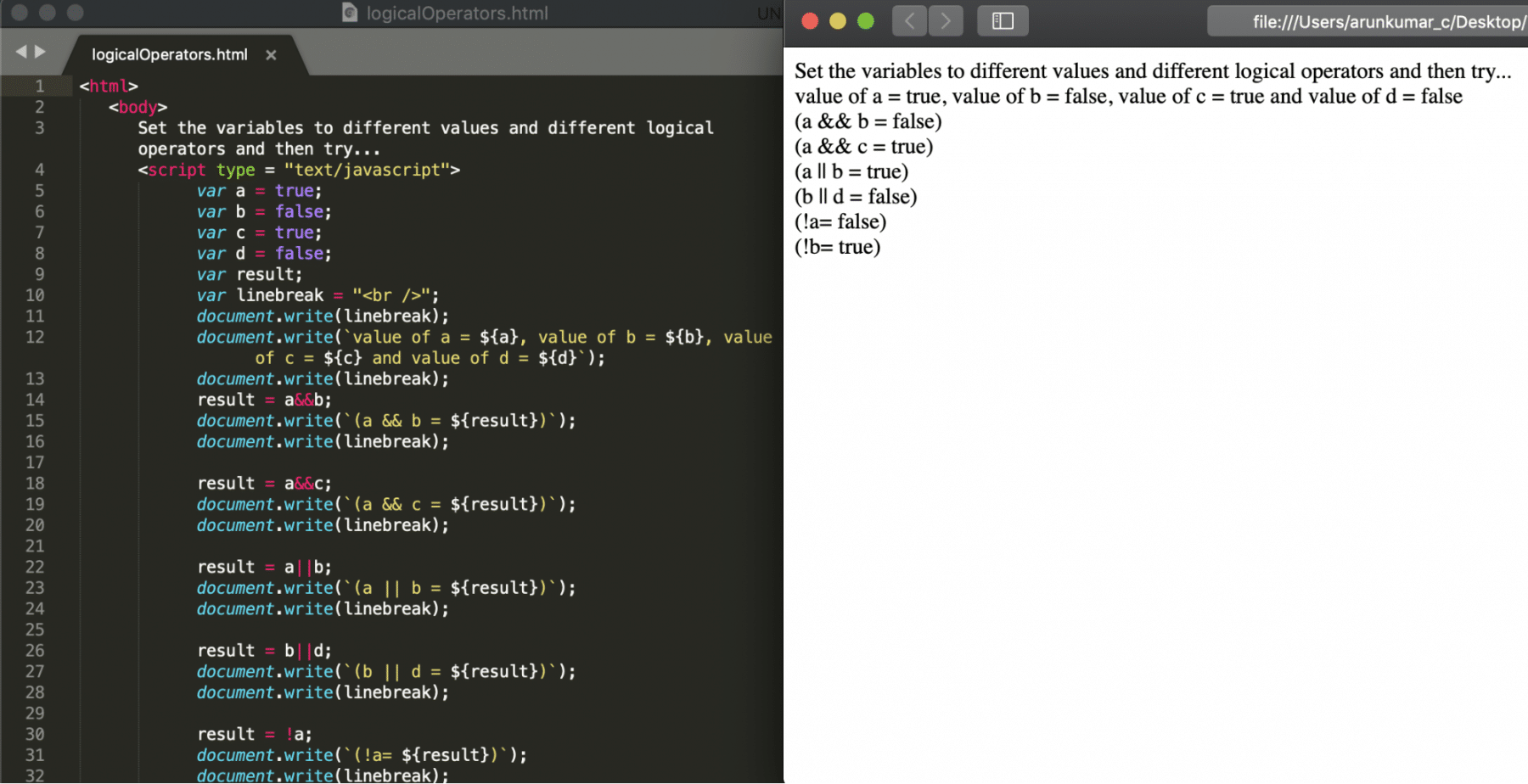### Bitwise JavaScript Operators:

Bitwise Operators are similar to logical operators except that they work on the bit level rather than decimal, hexadecimal, or octal numbers. Additionally, Bit-level programming only deals with 0 and 1. Moreover, they are used in numerical computations to make the calculation process faster.

JavaScript supports the following bitwise operators:

Name Operator Syntax Example
Bitwise AND & output = var1&var2; Output = 2&3;;
Bitwise OR | Output = var1|var2; Output = 3|2;
Bitwise XOR ^ Output = var1^var2; Output = 3^2;
Bitwise NOT ~ Output = ~var2; Output = ~5;
Left Shift << Output = var1< Output = 5<<1;
Right Shift >> Output = var1>>var2; Output = 4>>1;

Now let's understand the above mentioned Bitwise operators in detail:

• Bitwise AND ( & ): This operator performs ‘AND’ operation on each bit of its operands. E.g., in the below code snippet, it is equal to the calculation where firstly, convert the values of 2 and 5 in binary format. Secondly, do AND(&) of each bit. Finally, convert the binary result to the decimal result. As none of the bits on the same position is 1, the final result is 0 for & operation of all the bits individually.
``````var a = 2;// on bit level 2 = 0010
var b = 5;// on bit level 5 = 0101
var c = a & b; //the value of c will be 0, since a & b is 0000 when performed an operation on bit a level
``````
• Bitwise OR ( | ): This operator performs ‘OR’ operation on each bit of its operands. E.g., in the below code snippet, it is equal to the calculation where firstly, convert the values of 2 and 5 in binary format. Secondly, do OR(|) of each bit. Lastly, convert the binary result to the decimal result. The binary result will be 0111, which converted to decimal will be equivalent to 7.
``````var a = 2; // on bit-level 2 = 0010
var b = 5; // on bit-level 5 = 0101
var c = a | b; //the value of c will be 7, since a |  b is 0111 when performed OR operation on a bit level

``````
• Bitwise XOR ( ^ ): This operator performs ‘XOR’ operation on each bit of its operands. The XOR means exclusive OR operation. Here, the result will be true only when either of the operands is true, but not both.
``````var a = 2;// on bit-level 2 = 0010
var b = 7; // on bit-level 7 = 0111
var c = a ^ b; //the value of c will be 5, since a^ b is 0101 when performed XOR operation on a bit level
``````
• Bitwise NOT ( ~ ): This operator will invert all the bits on the operand.
``````var a = 3;// on bit-level 2 = 0011
var c = ~b; //the value of c will be -4, since a & b is 1100 where last bit will represent a positive or negative value
``````
• Left Shift (<<): This operator moves all bits to the left side by as many numbers of places as mentioned in the operand. In addition to this, new bits fill with zero value.
``````var a = 3; // 3 = 0011
var b = a <<1; the value of c will be 6, since a<<1 is 0110

``````
• Right Shift (>>): This operator will move all the bits to the right side by as many numbers of places as mentioned in the operand. In addition to this, new bits fill with zero value.
``````var a = 3; // 3 = 0011
var b = a >>1; the value of c will be 1, since a<<1 is 0001

``````

Let’s create a simple example using all the above mentioned bitwise operators.

``````<html>

<body>

Set the variables to different values and different bitwise operators and then try...

<script type = "text/javascript">

var a = 2;

var b = 5;

var result;

var linebreak = "<br />";

document.write(linebreak);

document.write(`value of a = \${a}, value of b = \${b}`);

document.write(linebreak);

result = a&b;

document.write(`(a & b = \${result})`);

document.write(linebreak);

a = 2;

b = 5;

document.write(`value of a = \${a}, value of b = \${b}`);

document.write(linebreak);

result = a|b;

document.write(`(a | b = \${result})`);

document.write(linebreak);

a = 2;

b = 7;

document.write(`value of a = \${a}, value of b = \${b}`);

document.write(linebreak);

result = a^b;

document.write(`(a | b = \${result})`);

document.write(linebreak);

a = 3;

document.write(`value of a = \${a}`);

document.write(linebreak);

result = ~a;

document.write(`(~a = \${result})`);

document.write(linebreak);

a = 3;

document.write(`value of a = \${a}`);

document.write(linebreak);

result = a<<1;

document.write(`(a<<1 = \${result})`);

document.write(linebreak);

a = 3;

document.write(`value of a = \${a}`);

document.write(linebreak);

result = a>>1;

document.write(`(a>>1 = \${result})`);

document.write(linebreak);

</script>

</body>

</html>

``````

Save the file with name bitwiseOperators.html. After that, open it in any browser (Chrome, Firefox, or IE). It should show the output as: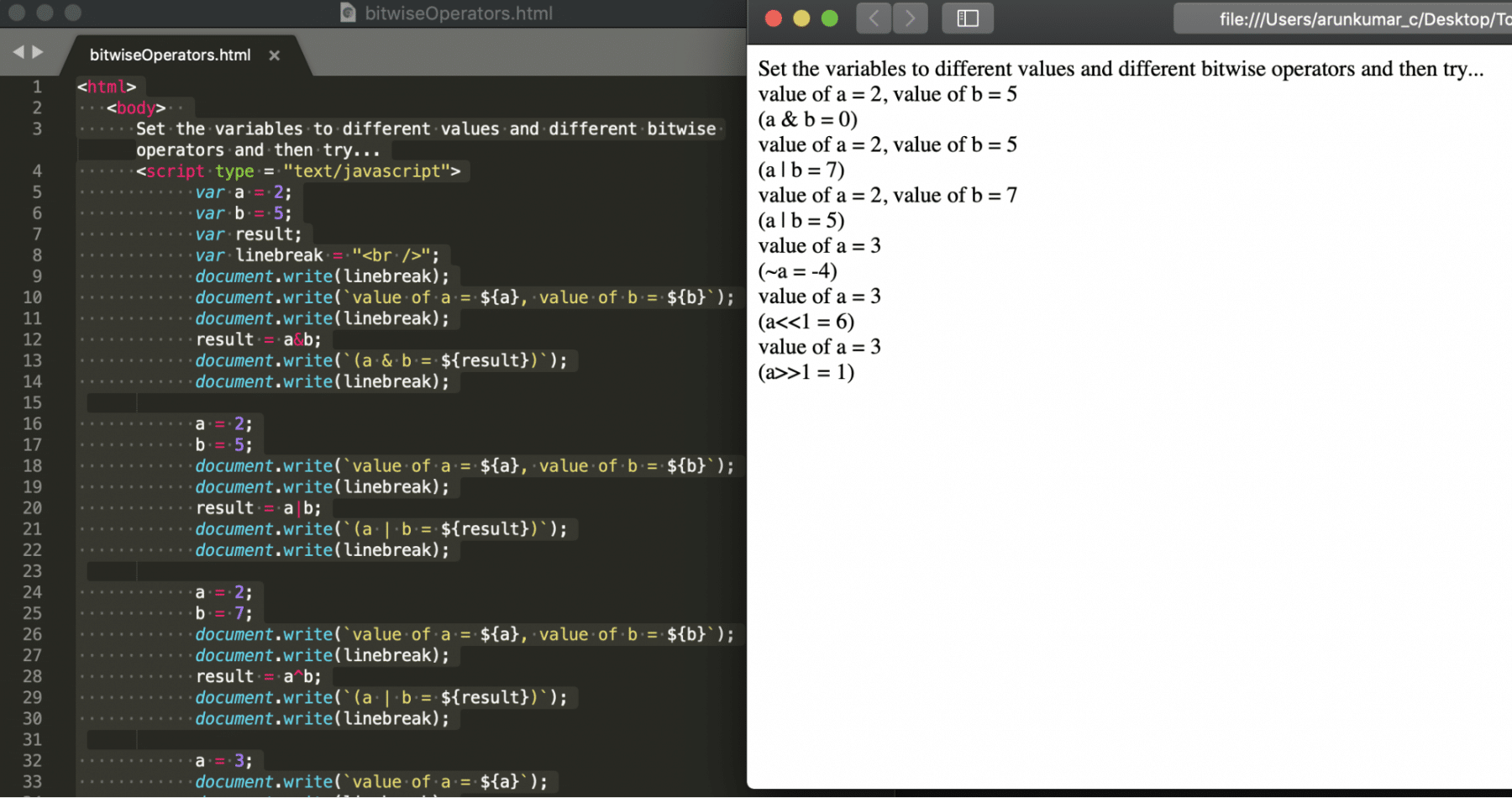### Miscellaneous JavaScript Operators:

JavaScript supports the number of other operators. We are going to discuss the primary two miscellaneous operators used most often.

Name Operator Syntax Example
Type of Operator typeof output = typeof var2; Output = typeof 3;
Conditional Operator ?: Output = condition?trueStat:falseStat; Output = 3<4?’greater’:’lesser’;

Now let's understand the above mentioned Miscellaneous operators in detail:

• typeOf Operator: The first operator is typeOf Operator. This operator is a unary operator. Additionally, it provides the data type of an operand.
``````var a = 50;
var result = typeof a; // this value of result will be number

``````
• Conditional Operator (?:)The second operator is a conditional operator. It is the only ternary operator (three operands) in JavaScript. In other words, this operator has three operands. First goes before the '?.' Second goes between the '?' and the ':.' Third goes after the ':.' If Condition is true? Then value A: Otherwise, value B.
``````var a = 50;
var result = (typeof a == “number”) ? “ a is of number type” : “a is of string type”; // this will return a is of number type
``````

Let’s create a simple example using above all the miscellaneous operators.

``````<html>

<body>

Set the variables to different values and different miscellaneous operators and then try...

<script type = "text/javascript">

var a = 2;

var result;

var linebreak = "</br>"

document.write(linebreak);

document.write(`value of a = \${a}`);

document.write(linebreak);

result = (typeof a == "number"? "a is of number type":"a is of String type");

document.write(`(\${result})`);

document.write(linebreak);

</script>

</body>

</html>

``````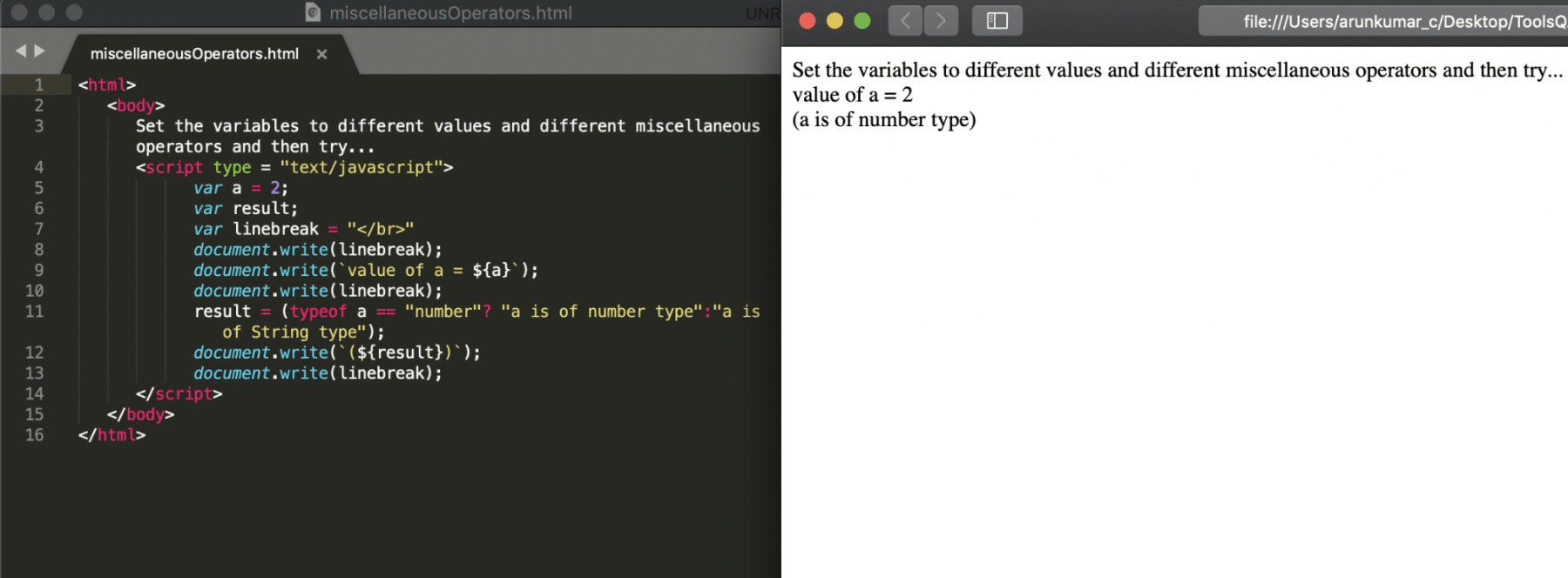## Key Takeaways

• JavaScript provides Arithmetic Operators such as +, -, *, /, %,++, - - . Additionally, they take numerical values as their operands and return a single numerical value.
• Moreover, it provides Assignment Operators such as =, +=, -=, *=, /=, %=. Additionally, they assign the right-hand side values after needed calculations to the left-hand-side variable.
• In addition to the above, the JavaScript provides Comparison Operators such as ==, !=, >, >=, <, <= .They are compare the values of two operands.
• JavaScript provides Logical Operators such as &&, ||, ! which control the program flow. Additionally, the majority of conditional statements use them. (Note: We will discuss the conditional statements in the next tutorial)
• In addition to above, it provides Bitwise Operators such as &, |, ^, ~, <<, >>. They are similar to logical operators, but they work directly at the bit level.

Conclusively, let's move to the next article. It will help us understand the next important construct of the JavaScript, Conditional Statements.